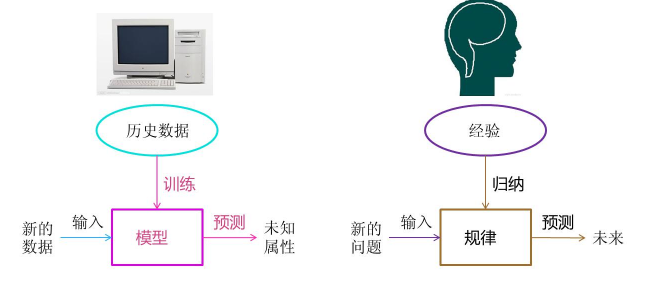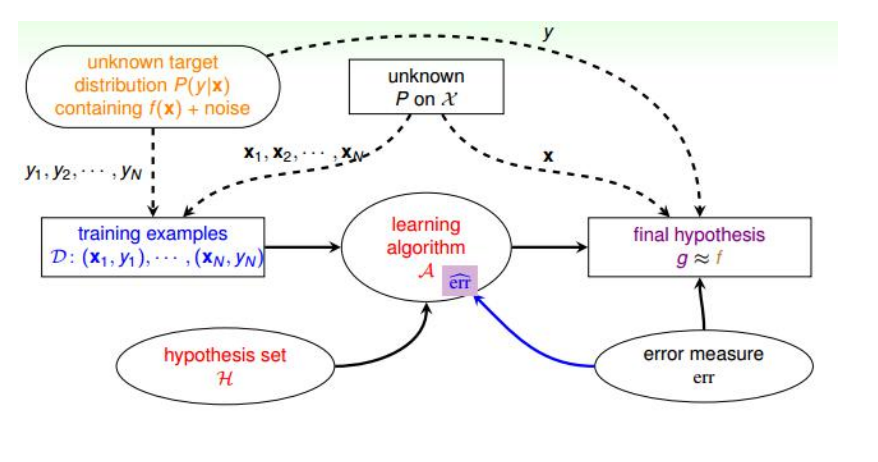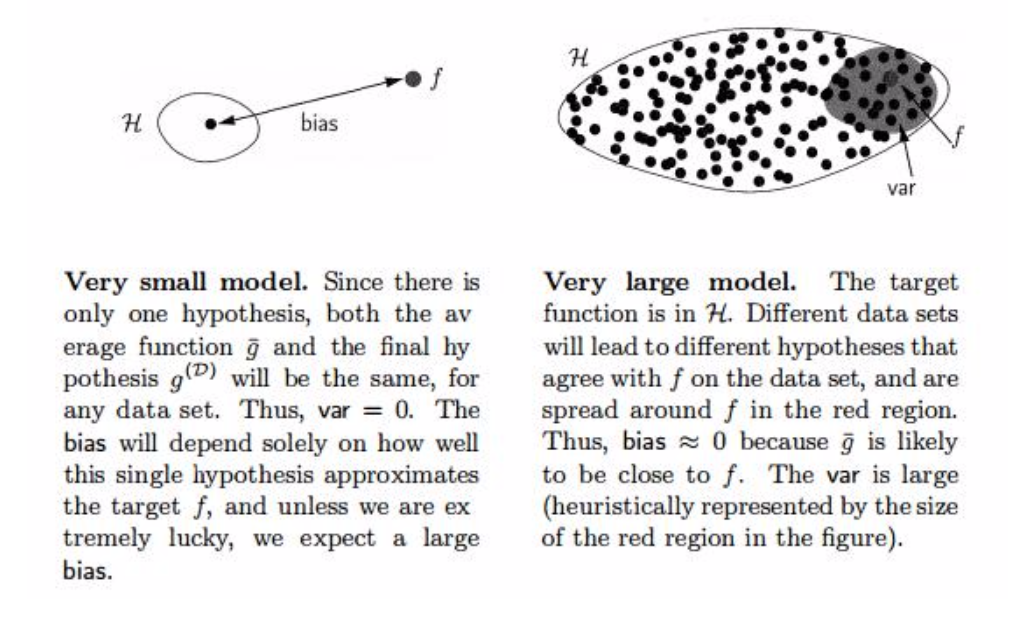# Note of PRML(1)

## The background of machine learning

Posted by GwanSiu on September 1, 2017

# 1. Introduction

PMRL第一章简单讲述了三方面的知识:(1).Machine Learning的基本知识,包括基本名词定义，模型选择以及维度灾难；(2).贝叶斯理论和决策理论；(3).信息理论与Machine Learning的联系。

# 2. The Background of Machine Learning

## 2.1 The definition of machine Learning$P(X|\Theta)=\frac{P(X)P(\Theta|X)}{P{\Theta}} \tag{1}$

PRML这一本书，则是从贝叶斯理论的框架下讨论一些列的机器学习问题。

## 2.2 The Type of Machine Learning

1. 从输出空间$y$的类型上分类(即:任务分类)：
若$y$是离散空间(Discrete Set)，则该任务为分类(Classification)
若$y$是连续空间(Continuous Set)，则该任务为回归(Regresssion)
1. 从学习的目的上分类： 监督式学习(Supervised Learning): 有标签式学习，数据与标签成对$(x_{n},y_{n})$存在。 非监督式学习(Unsupervised Learning):无标签式学习，只有数据$X=(x_{1},…,x_{n})$,无监督式学习的目的是将相似的数据聚类(Cluster), 本质上是学习潜在的概率分布(dense estimation)。
半监督式学习(Semi-supervised Learning):有标签的数据和无标签的数据都有，利用有标签数据辅助学习，从而学到无标签数据潜在的概率分布。

## 2.3 The Feasibility of Machine Learning

1. 机器学习要解决的根本问题
• 机器学习的必要不充分条件:在训练集的数据和测试集的数据需来源同一分布或者两个相近的分布的前提下,且要做到以下两点：
• (1) 保证训练误差$E_{train}$很小(训练过程)。
• (2) 使得测试误差要逼近训练误差的值$E_{test}\approx E_{train}$(测试过程，保证了模型的泛化能力)，这样就保证了测试误差也很小。

$E_{test}\leqslant E_{train} + \sqrt(\frac{8}{N}\text{In}\frac{4m_{H}(2N)}{\delta})\leqslant E_{train} + \sqrt(\frac{8}{N}\text{In}\frac{4(2N)^{d_{vc}}}{\delta} \tag{2}$ $E_{test}\leqslant E_{train} + \Omega(N,H,\delta) \tag{3}$

VC维度的讨论

1. 当数据样本$N$一定时，若d_{vc}(H) 很高，说明学习到的模型复杂度高，这会导致$E_{train}$很小，但$\Omega(N,H,\delta)$很大便导致$E_{test} < E_{train}$, 条件(2)不能满足。这属于过拟合现象。从上式可知解决过拟合现象有两种方法，一是增大样本量$N$,二是减少vc维度，$d_{vc}$,即:Regulization.

2. 当数据样本$N$一定时，若d_{vc}(H)很小，说明学习到的模型复杂度低，这会使得$E_{train}$很高，即使条件(2)可以满足:$E_{test}\approx E_{train}$，但$E_{test}$很大，学习效果很差。这属于欠拟合现象，解决方法是增大vc维度。

$\Vert g(x,w)-f(x) \Vert^{2}$

$E_{test}[g^{D}(x)] = E_{x}[\lVert g^{D}(x)-f(c)\rVert^{2}] \tag{4}$ \begin{aligned} E_{D}[E_{test}[g^{D}]] &= E_{D}[E_{x}[\lVert g^{D}(x)-f(x) \rVert^2]] \\ &=E_{x}[E_{D}[g^{D}(x)^{2}]-2\hat{g}^{D}(x)f(x)+f^{2}(x)] \\ &=E_{x}[E_{D}[g^{D}(x)^{2}]-\hat{g}^{D}(x)^{2}+\hat{g}^{D}(x)^{2}-2\hat{g}^{D}(x)f(x)+f^{2}(x)] \\ &=E_{x}[E_{D}[g^{D}(x)^{2}]-\hat{g}^{D}(x)^{2}]+E_{x}[(\hat{g}^{D}(x)-f(x))^{2}] \end{aligned}

$\text{Var(x)}=E_{D}[g^{D}(x)^{2}]-\hat{g}^{D}(x)^{2}$.

$E_{D}[E_{test}[g^{D}]]=\text{bias(x)}+\text{Var{x}} \tag{6}$bias很大，说明所构造的函数空间的均值点$E[g^{D}(x)]$离&f(x)$距离远，模型因此欠拟合；Variance很大，说明所构造的函数空间虽然包含$f(x)\$，但是空间很大，离散程度高，模型容易过拟合。